Main Content

# comm.CarrierSynchronizer

Compensate for carrier frequency offset

## Description

The `comm.CarrierSynchronizer` System object™ compensates for carrier frequency and phase offsets in signals that use single-carrier modulation schemes. The carrier synchronizer algorithm is compatible with BPSK, QPSK, OQPSK, 8-PSK, PAM, and rectangular QAM modulation schemes.

Note

• This System object does not resolve phase ambiguities created by the synchronization algorithm. As indicated in this table, the potential phase ambiguity introduced by the synchronizer depends on the modulation type:

ModulationPhase Ambiguity (degrees)
`'BPSK'` or `'PAM'`0, 180
`'OQPSK'`, `'QPSK'`, or `'QAM'`0, 90, 180, 270
`'8PSK'`0, 45, 90, 135, 180, 225, 270, 315

The Examples demonstrate carrier synchronization and resolution of phase ambiguity.

• For best results, apply carrier synchronization to non-oversampled signals, as demonstrated in Correct Phase and Frequency Offset for 16-QAM Using Coarse and Fine Synchronization.

To compensate for frequency and phase offsets in signals that use single-carrier modulation schemes:

1. Create the `comm.CarrierSynchronizer` object and set its properties.

2. Call the object, as if it were a function.

To learn more about how System objects work, see What Are System Objects?.

## Creation

### Syntax

``carrSynch = comm.CarrierSynchronizer``
``carrSynch = comm.CarrierSynchronizer(Name,Value)``

### Description

````carrSynch = comm.CarrierSynchronizer` creates a System object that compensates for carrier frequency offset and phase offset in signals that use single-carrier modulation schemes.```

example

``` `carrSynch = comm.CarrierSynchronizer(Name,Value)` sets properties using one or more name-value pairs. Enclose each property name in quotes.```

## Properties

expand all

Unless otherwise indicated, properties are nontunable, which means you cannot change their values after calling the object. Objects lock when you call them, and the `release` function unlocks them.

If a property is tunable, you can change its value at any time.

For more information on changing property values, see System Design in MATLAB Using System Objects.

Modulation type, specified as `'QAM'`, `'8PSK'`, `'BPSK'`, `'OQPSK'`, `'PAM'`, or `'QPSK'`.

Example: `comm.CarrierSynchronizer('Modulation','QPSK')` creates a carrier synchronizer System object to use with a QPSK modulated signal.

Tunable: No

Modulation phase offset method, specified as `'Auto'` or `'Custom'`.

• `'Auto'` — Apply the traditional offset for the specified modulation type.

ModulationPhase Offset (radians)
`'BPSK'`, `'QAM'`, or `'PAM'`0
`'OQPSK'` or `'QPSK'`π/4
`'8PSK'`π/8
• `'Custom'` — Specify a user-defined phase offset with the CustomPhaseOffset property.

Tunable: Yes

Custom phase offset in radians, specified as a scalar.

#### Dependencies

This property applies when the ModulationPhaseOffset property is set to `'Custom'`.

Data Types: `double`

Number of samples per symbol, specified as a positive integer.

Tunable: Yes

Data Types: `double`

Damping factor of the loop, specified as a positive scalar.

Tunable: Yes

Data Types: `double`

Normalized bandwidth of the loop, specified as a scalar in the range (0,1]. The loop bandwidth is normalized by the sample rate of the synchronizer.

Decreasing the loop bandwidth reduces the synchronizer convergence time but also reduces the pull-in range of the synchronizer.

Tunable: Yes

Data Types: `double`

## Usage

### Syntax

``[outSig,phErr] = carrSynch(inSig)``

### Description

example

````[outSig,phErr] = carrSynch(inSig)` compensates for frequency offset and phase offset in the input signal. This System object returns a compensated output signal and an estimate of the phase error.```

### Input Arguments

expand all

Input signal, specified as a complex scalar or a column vector of complex values.

Data Types: `double` | `single`
Complex Number Support: Yes

### Output Arguments

expand all

Output signal, returned as a scalar or column vector with the same data type and length as `inSig`. The output signal adjusts the input signal compensating for carrier frequency and phase offsets in signals that use single-carrier modulation schemes.

Phase error estimate in radians, returned as a scalar or column vector with the same length as `inSig`.

## Object Functions

To use an object function, specify the System object as the first input argument. For example, to release system resources of a System object named `obj`, use this syntax:

`release(obj)`

expand all

 `info` Characteristic information about carrier synchronizer `clone` Create duplicate System object `isLocked` Determine if System object is in use
 `step` Run System object algorithm `release` Release resources and allow changes to System object property values and input characteristics `reset` Reset internal states of System object

## Examples

collapse all

Correct phase and frequency offsets of a QPSK signal passed through an AWGN channel. Using preambles, resolve phase ambiguity.

Define the simulation parameters.

```M = 4; % Modulation order rng(1993) % For repeatable results barker = comm.BarkerCode(... 'Length',13,'SamplesPerFrame',13); % For preamble msgLen = 1e4; numFrames = 10; frameLen = msgLen/numFrames;```

Add preambles to each frame, which are used later when performing phase ambiguity resolution. Generate random data symbols, and apply QPSK modulation.

```preamble = (1+barker())/2; % Length 13, unipolar data = zeros(msgLen,1); for idx = 1 : numFrames payload = randi([0 M-1],frameLen-barker.Length,1); data((idx-1)*frameLen + (1:frameLen)) = [preamble; payload]; end modSig = pskmod(data,4,pi/4);```

Create a `comm.PhaseFrequencyOffset` System object™ to introduce phase and frequency offsets to the modulated input signal. Set the phase offset to `45` degrees, frequency offset to 1 kHz, and sample rate to 10 kHz. The frequency offset is set to 1% of the sample rate.

```pfo = comm.PhaseFrequencyOffset('PhaseOffset',45, ... 'FrequencyOffset',1e4,'SampleRate',1e6);```

Create a carrier synchronizer System object to use for correcting the phase and frequency offsets with samples per symbol set to 1.

```carrierSync = comm.CarrierSynchronizer( ... 'SamplesPerSymbol',1,'Modulation','QPSK');```

Apply phase and frequency offsets using the `pfo` System object, and then pass the signal through an AWGN channel to add white Gaussian noise.

```modSigOffset = pfo(modSig); rxSig = awgn(modSigOffset,12);```

Display the scatter plot of the received signal. The data appear in a circle instead of being grouped around the reference constellation points due to the frequency offset.

`scatterplot(rxSig)`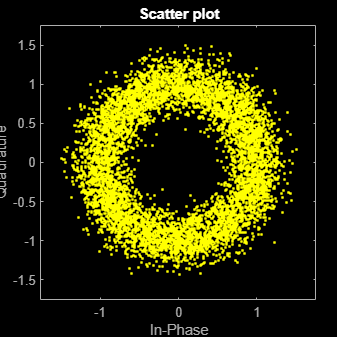Use the `carrierSync` System object to correct the phase and frequency offset in the received signal.

`syncSignal = carrierSync(rxSig);`

Use a constellation diagram to display the first and last 1000 symbols of the synchronized signal. Before convergence of the synchronizer loop, the plotted symbols are not grouped around the reference constellation points. After convergence, the plotted symbols are grouped around the reference constellation points.

```constDiag = comm.ConstellationDiagram( ... 'SymbolsToDisplaySource','Property','SymbolsToDisplay',300, ... 'ChannelNames',{'Before convergence','After convergence'},'ShowLegend',true, ... 'Position',[400 400 400 400]); constDiag([syncSignal(1:1000) syncSignal(9001:10000)]);```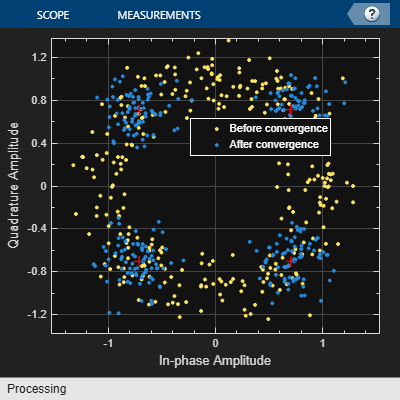Demodulate the synchronized signal. Compute and display the total bit errors and BER.

```syncData = pskdemod(syncSignal,4,pi/4); [syncDataTtlErr,syncDataBER] = biterr(data(6000:end),syncData(6000:end))```
```syncDataTtlErr = 3990 ```
```syncDataBER = 0.4986 ```

Phase ambiguity in the received signal might cause bit errors. Using the preamble, determine phase ambiguity. Remove this phase ambiguity from the synchronized signal to reduce bit errors.

```idx = 9000 + (1:barker.Length); phOffset = angle(modSig(idx) .* conj(syncSignal(idx))); phOffset = round((2/pi) * phOffset); % -1, 0, 1, +/-2 phOffset(phOffset==-2) = 2; % Prep for mean operation phOffset = mean((pi/2) * phOffset); % -pi/2, 0, pi/2, or pi disp(['Estimated mean phase offset = ',num2str(phOffset*180/pi),' degrees'])```
```Estimated mean phase offset = 180 degrees ```
`resPhzSig = exp(1i*phOffset) * syncSignal;`

Demodulate the signal after resolving the phase ambiguity. Recompute and display the updated total bit errors and BER. Removing the phase ambiguity reduces the BER dramatically.

```resPhzData = pskdemod(resPhzSig,4,pi/4); [resPhzTtlErr, resPhzBER] = biterr(data(6000:end),resPhzData(6000:end))```
```resPhzTtlErr = 403 ```
```resPhzBER = 0.0504 ```

Estimate the frequency offset introduced into a noisy 8-PSK signal using a carrier synchronizer System object™.

Define the simulation parameters.

```M = 8; % Modulation order fs = 1e6; % Sample rate (Hz) foffset = 1000; % Frequency offset (Hz) phaseoffset = 15; % Phase offset (deg) snrdb = 20; % Signal-to-noise ratio (dB)```

Create a `comm.PhaseFrequencyOffset` System object to introduce phase and frequency offsets to a modulated signal.

```pfo = comm.PhaseFrequencyOffset('PhaseOffset',phaseoffset, ... 'FrequencyOffset',foffset,'SampleRate',fs);```

Create a carrier synchronizer System object to use for correcting the phase and frequency offsets. Set the `Modulation` property to `8PSK`.

`carrierSync = comm.CarrierSynchronizer('Modulation','8PSK');`

Generate random data and apply 8-PSK modulation.

```data = randi([0 M-1],5000,1); modSig = pskmod(data,M,pi/M);```

Apply phase and frequency offsets using the pfo System object, and pass the signal through an AWGN channel to add Gaussian white noise.

```modSigOffset = pfo(modSig); rxSig = awgn(modSigOffset,snrdb);```

Use the carrier synchronizer to estimate the phase offset of the received signal.

`[~,phError] = carrierSync(rxSig);`

Determine the frequency offset by using the `diff` function to compute an approximate derivative of the phase error. The derivative must be scaled by $2\pi$ because the phase error is measured in radians.

`estFreqOffset = diff(phError)*fs/(2*pi);`

Plot the running mean of the estimated frequency offset. After the synchronizer converges to a solution, the mean value of the estimate is approximately equal to the input frequency offset value of 1000 Hz.

```rmean = cumsum(estFreqOffset)./(1:length(estFreqOffset))'; plot(rmean) xlabel('Symbols') ylabel('Estimated Frequency Offset (Hz)') grid```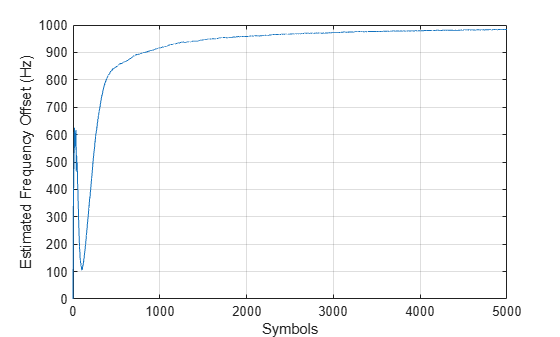Compensation of significant phase and frequency offsets for a 16-QAM signal in an AWGN channel is accomplished in two steps. First, correct the coarse frequency offset using the estimate provided by the coarse frequency compensator, and then fine-tune the correction using carrier synchronization. Because of the coarse frequency correction, the carrier synchronizer converges quickly even though the normalized bandwidth is set to a low value. Lower normalized bandwidth values enable better correction for small residual carrier offsets. After applying phase and frequency offset corrections to the received signal, resolve phase ambiguity using the preambles.

Define the simulation parameters.

```fs = 10000; % Sample rate (Hz) sps = 4; % Samples per symbol M = 16; % Modulation order k = log2(M); % Bits per symbol rng(1996) % Set seed for repeatable results barker = comm.BarkerCode(... 'Length',13,'SamplesPerFrame',13); % For preamble msgLen = 1e4; numFrames = 10; frameLen = msgLen/numFrames; ```

Generate data payloads and add the preamble to each frame. The preamble is later used for phase ambiguity resolution.

```preamble = (1+barker())/2; % Length 13, unipolar data = zeros(msgLen, 1); for idx = 1 : numFrames payload = randi([0 M-1],frameLen-barker.Length,1); data((idx-1)*frameLen + (1:frameLen)) = [preamble; payload]; end```

Create a System object for the transmit pulse shape filtering, the receive pulse shape filtering, the QAM coarse frequency compensation, the carrier synchronization, and a constellation diagram.

```txFilter = comm.RaisedCosineTransmitFilter( ... 'OutputSamplesPerSymbol',sps); rxFilter = comm.RaisedCosineReceiveFilter(... 'InputSamplesPerSymbol',sps,'DecimationFactor',sps); coarse = comm.CoarseFrequencyCompensator('SampleRate',fs, ... 'FrequencyResolution',10); fine = comm.CarrierSynchronizer( ... 'DampingFactor',0.4,'NormalizedLoopBandwidth',0.001, ... 'SamplesPerSymbol',1,'Modulation','QAM'); axislimits = [-6 6]; constDiagram = comm.ConstellationDiagram('ReferenceConstellation',qammod(0:M-1,M), ... 'ChannelNames',{'Before convergence','After convergence'}, ... 'ShowLegend',true,'XLimits',axislimits,'YLimits',axislimits);```

Also create a System object for the AWGN channel, and the phase and frequency offset to add impairments to the signal. A phase offset greater than 90 degrees is added to induce a phase ambiguity that results in a constellation quadrant shift.

```ebn0 = 8; freqoffset = 110; phaseoffset = 110; awgnChannel = comm.AWGNChannel('EbNo',ebn0, ... 'BitsPerSymbol',k,'SamplesPerSymbol',sps); pfo = comm.PhaseFrequencyOffset('FrequencyOffset',freqoffset, ... 'PhaseOffset',phaseoffset,'SampleRate',fs);```

Generate random data symbols, apply 16-QAM modulation, and pass the modulated signal through the transmit pulse shaping filter.

```txMod = qammod(data,M); txSig = txFilter(txMod);```

Apply phase and frequency offsets using the `pfo` System object, and then pass the signal through an AWGN channel to add white Gaussian noise.

```txSigOffset = pfo(txSig); rxSig = awgnChannel(txSigOffset);```

The coarse frequency compensator System object provides a rough correction for the frequency offset. For the conditions in this example, correcting the frequency offset of the received signal correction to within 10 Hz of the transmitted signal is sufficient.

`syncCoarse = coarse(rxSig);`

Pass the signal through the receive pulse shaping filter, and apply fine frequency correction.

`rxFiltSig = fine(rxFilter(syncCoarse));`

Display the constellation diagram of the first and last 1000 symbols in the signal. Before convergence of the synchronization loop, the spiral nature of the diagram indicates that the frequency offset is not corrected. After the carrier synchronizer has converged to a solution, the symbols are aligned with the reference constellation.

`constDiagram([rxFiltSig(1:1000) rxFiltSig(9001:end)])`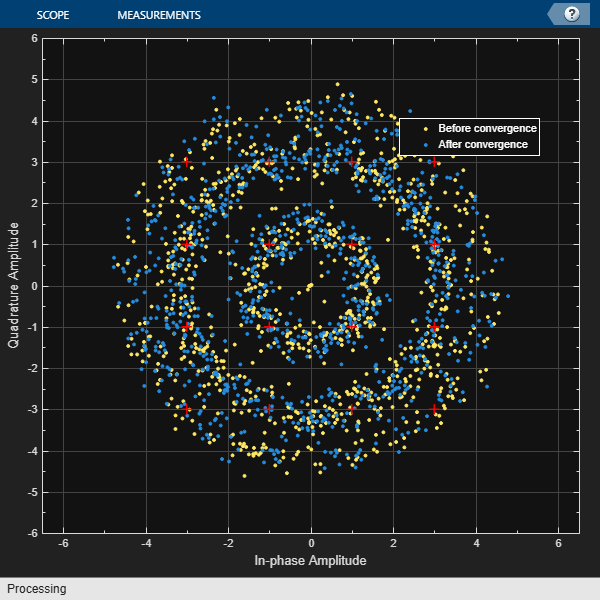Demodulate the signal. Account for the signal delay caused by the transmit and receive filters to align the received data with the transmitted data. Compute and display the total bit errors and BER. When checking the bit errors, use the later portion of the received signal to be sure the synchronization loop has converged.

```rxData = qamdemod(rxFiltSig,M); delay = (txFilter.FilterSpanInSymbols + rxFilter.FilterSpanInSymbols) / 2; idxSync = 2000; % Check BER for the received signal after the synchronization loop has converged [syncDataTtlErr,syncDataBER] = biterr(data(idxSync:end-delay),rxData(idxSync+delay:end))```
```syncDataTtlErr = 16116 ```
```syncDataBER = 0.5042 ```

Depending on the random data used, there may be bit errors resulting from phase ambiguity in the received signal after the synchronization loop converges and locks. In this case, you can use the preamble to determine and then remove the phase ambiguity from the synchronized signal to reduce bit errors. If phase ambiguity is minimal, the number of bit errors may be unchanged.

```idx = 9000 + (1:barker.Length); phOffset = angle(txMod(idx) .* conj(rxFiltSig(idx+delay))); phOffsetEst = mean(phOffset); disp(['Phase offset = ',num2str(rad2deg(phOffsetEst)),' degrees'])```
```Phase offset = -90.1401 degrees ```
`resPhzSig = exp(1i*phOffsetEst) * rxFiltSig;`

Demodulate the signal after resolving the phase ambiguity. Recompute the total bit errors and BER.

```resPhzData = qamdemod(resPhzSig,M); [resPhzTtlErr,resPhzBER] = biterr(data(idxSync:end-delay),resPhzData(idxSync+delay:end))```
```resPhzTtlErr = 5 ```
```resPhzBER = 1.5643e-04 ```

Model channel impairments such as timing phase offset, carrier frequency offset, and carrier phase offset for a minimum shift keying (MSK) signal. Use `comm.MSKTimingSynchronizer` and `comm.CarrierSynchronizer` System objects to synchronize such signals at the receiver. The MSK timing synchronizer recovers the timing offset, while a carrier synchronizer recovers the carrier frequency and phase offsets.

Initialize system variables by running the MATLAB script `configureMSKSignalRecoveryEx`. Define the logical control variable `recoverTimingPhase` to enable timing phase recovery, and `recoverCarrier` to enable carrier frequency and phase recovery.

```configureMSKSignalRecoveryEx; recoverTimingPhase = true; recoverCarrier = true; ```

Modeling Channel Impairments

Specify the sample delay, `timingOffset`, that the channel model applies. Create a variable fractional delay object to introduce the timing delay to the transmitted signal.

```timingOffset = 0.2; varDelay = dsp.VariableFractionalDelay; ```

Create a `comm.PhaseFrequencyOffset` System object to introduce carrier phase and frequency offsets to a modulated signal. Because the MSK modulator upsamples the transmitted symbols, set the `SampleRate` property to the ratio of the `samplesPerSymbol` and the sample time, `Ts`.

```freqOffset = 50; phaseOffset = 30; pfo = comm.PhaseFrequencyOffset(... 'FrequencyOffset',freqOffset, ... 'PhaseOffset',phaseOffset, ... 'SampleRate',samplesPerSymbol/Ts); ```

Create a `comm.AWGNChannel` System object to add white Gaussian noise to the modulated signal. The noise power is determined by the `EbNo` property, that is the bit energy to noise power spectral density ratio. Because the MSK modulator generates symbols with 1 Watt of power, set the signal power property of the AWGN channel System object to 1.

```EbNo = 20 + 10*log10(samplesPerSymbol); chAWGN = comm.AWGNChannel(... 'NoiseMethod','Signal to noise ratio (Eb/No)', ... 'EbNo',EbNo,... 'SignalPower',1, ... 'SamplesPerSymbol',samplesPerSymbol); ```

Timing Phase, Carrier Frequency, and Carrier Phase Synchronization

Create an MSK timing synchronizer to recover symbol timing phase using a fourth-order nonlinearity method.

```timeSync = comm.MSKTimingSynchronizer(... 'SamplesPerSymbol',samplesPerSymbol, ... 'ErrorUpdateGain',0.02); ```

Create a carrier synchronizer to recover both carrier frequency and phase. Because the MSK constellation is QPSK with a 0-degree phase offset, set the `comm.CarrierSynchronizer` accordingly.

```phaseSync = comm.CarrierSynchronizer(... 'Modulation','QPSK', ... 'ModulationPhaseOffset','Custom', ... 'CustomPhaseOffset',0, ... 'SamplesPerSymbol',1); ```

Stream Processing Loop

The simulation modulates data using MSK modulation. The modulated symbols pass through the channel model, which applies timing delay, carrier frequency and phase shift, and additive white Gaussian noise. The receiver performs timing phase and carrier frequency and phase recovery. Finally, the signal symbols are demodulated and the bit error rate is calculated. The `plotResultsMSKSignalRecoveryEx` script generates scatter plots in this order to show these effects:

1. Channel impairments

2. Timing synchronization

3. Carrier synchronization

At the end of the simulation, the example displays the timing phase, frequency, and phase estimates as a function of simulation time.

```for p = 1:numFrames %------------------------------------------------------------------------ % Generate and modulate data %------------------------------------------------------------------------ txBits = randi([0 1],samplesPerFrame,1); txSym = modem(txBits); %------------------------------------------------------------------------ % Transmit through channel %------------------------------------------------------------------------ % % Add timing offset rxSigTimingOff = varDelay(txSym,timingOffset*samplesPerSymbol); % % Add carrier frequency and phase offset rxSigCFO = pfo(rxSigTimingOff); % % Pass the signal through an AWGN channel rxSig = chAWGN(rxSigCFO); % % Save the transmitted signal for plotting plot_rx = rxSig; % %------------------------------------------------------------------------ % Timing recovery %------------------------------------------------------------------------ if recoverTimingPhase % Recover symbol timing phase using fourth-order nonlinearity % method [rxSym,timEst] = timeSync(rxSig); % Calculate the timing delay estimate for each sample timEst = timEst(1)/samplesPerSymbol; else % Do not apply timing recovery and simply downsample the received % signal rxSym = downsample(rxSig,samplesPerSymbol); timEst = 0; end % Save the timing synchronized received signal for plotting plot_rxTimeSync = rxSym; %------------------------------------------------------------------------ % Carrier frequency and phase recovery %------------------------------------------------------------------------ if recoverCarrier % The following script applies carrier frequency and phase recovery % using a second order phase-locked loop (PLL), and removes phase ambiguity [rxSym,phEst] = phaseSync(rxSym); removePhaseAmbiguityMSKSignalRecoveryEx; freqShiftEst = mean(diff(phEst)/(Ts*2*pi)); phEst = mod(mean(phEst),360); % in degrees else freqShiftEst = 0; phEst = 0; end % Save the phase synchronized received signal for plotting plot_rxPhSync = rxSym; %------------------------------------------------------------------------ % Demodulate the received symbols %------------------------------------------------------------------------ rxBits = demod(rxSym); %------------------------------------------------------------------------ % Calculate the bit error rate %------------------------------------------------------------------------ errorStats = BERCalc(txBits,rxBits); %------------------------------------------------------------------------ % Plot results %------------------------------------------------------------------------ plotResultsMSKSignalRecoveryEx; end ```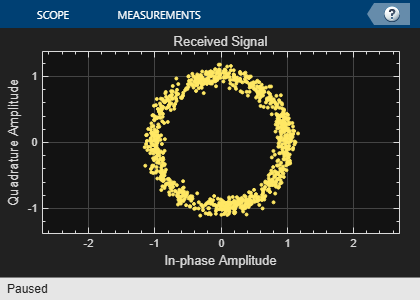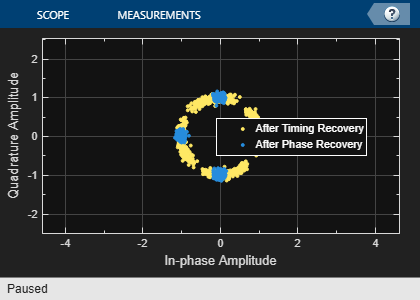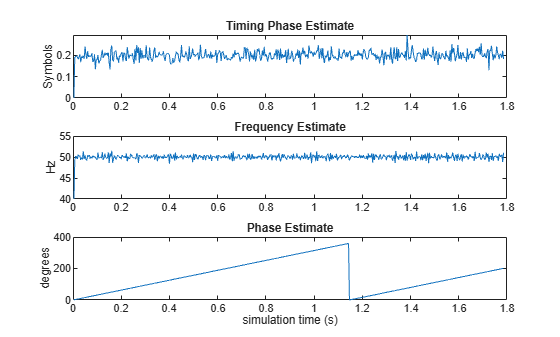Display the bit error rate and the total number of symbols processed by the error rate calculator.

```BitErrorRate = errorStats(1) TotalNumberOfSymbols = errorStats(3) ```
```BitErrorRate = 4.0001e-06 TotalNumberOfSymbols = 499982 ```

Conclusion and Further Experimentation

The recovery algorithms are demonstrated by using constellation plots taken after timing, carrier frequency, and carrier phase synchronization.

Open the script to create a writable copy of this example and its supporting files. Then, to show the effects of the recovery algorithms, you can enable and disable the logical control variables `recoverTimingPhase` and `recoverCarrier` and rerun the simulation.

Appendix

This example uses these scripts:

• `configureMSKSignalRecoveryEx`

• `plotResultsMSKSignalRecoveryEx`

• `removePhaseAmbiguityMSKSignalRecoveryEx`

## Algorithms

The `comm.CarrierSynchronizer` System object is a closed-loop compensator that uses the PLL-based algorithm described in . The output of the synchronizer, yn, is a frequency-shifted version of the complex input signal, xn, for the nth sample. The synchronizer output is

`${y}_{\text{n}}={x}_{\text{n}}{e}^{i{\lambda }_{\text{n}}}\text{\hspace{0.17em}},$`

where λn is the output of the direct digital synthesizer (DDS). The DDS is the discrete-time version of a voltage-controlled oscillator and is a core component of discrete-time phase locked loops. In the context of this System object, the DDS works as an integration filter.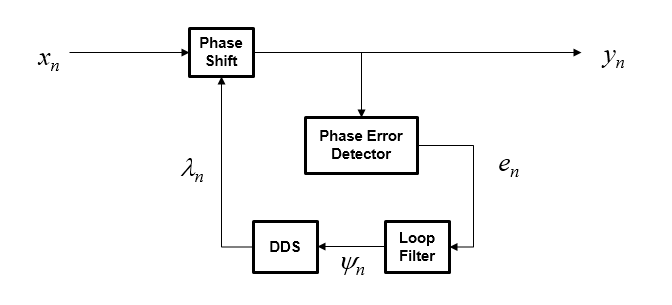To correct for the frequency offset, first the algorithm determines the phase error, en. The value of the phase error depends on the modulation scheme.

ModulationPhase Error
QAM or QPSK

${e}_{\text{n}}=\mathrm{sgn}\left(\mathrm{Re}\left\{{x}_{\text{n}}\right\}\right)×\mathrm{Im}\left\{{x}_{\text{n}}\right\}-\mathrm{sgn}\left(\mathrm{Im}\left\{{x}_{\text{n}}\right\}\right)×\mathrm{Re}\left\{{x}_{\text{n}}\right\}$

For a detailed description of this equation, see .

BPSK or PAM

${e}_{\text{n}}=\mathrm{sgn}\left(\mathrm{Re}\left\{{x}_{\text{n}}\right\}\right)×\mathrm{Im}\left\{{x}_{\text{n}}\right\}$

For a detailed description of this equation, see .

8-PSK

${e}_{\text{n}}=\left\{\begin{array}{c}\mathrm{sgn}\left(\mathrm{Re}\left\{{x}_{\text{n}}\right\}\right)×\mathrm{Im}\left\{{x}_{\text{n}}\right\}-\left(\sqrt{2}-1\right)\mathrm{sgn}\left(\mathrm{Im}\left\{{x}_{\text{n}}\right\}\right)×\mathrm{Re}\left\{{x}_{\text{n}}\right\}\text{,}\text{ }\text{for}\text{ }|\mathrm{Re}\left\{{x}_{\text{n}}\right\}|\ge |\mathrm{Im}\left\{{x}_{\text{n}}\right\}|\\ \left(\sqrt{2}-1\right)\mathrm{sgn}\left(\mathrm{Re}\left\{{x}_{\text{n}}\right\}\right)×\mathrm{Im}\left\{{x}_{\text{n}}\right\}-\mathrm{sgn}\left(\mathrm{Im}\left\{{x}_{\text{n}}\right\}\right)×\mathrm{Re}\left\{{x}_{\text{n}}\right\}\text{,}\text{ }\text{for}\text{ }|\mathrm{Re}\left\{{x}_{\text{n}}\right\}|<|\mathrm{Im}\left\{{x}_{\text{n}}\right\}|\end{array}$

For a detailed description of this equation, see .

OQPSK

${e}_{\text{n}}=\mathrm{sgn}\left(\mathrm{Re}\left\{{x}_{\text{n-SamplePerSymbol/2}}\right\}\right)×\mathrm{Im}\left\{{x}_{\text{n-SamplePerSymbol/2}}\right\}-\mathrm{sgn}\left(\mathrm{Im}\left\{{x}_{\text{n}}\right\}\right)×\mathrm{Re}\left\{{x}_{\text{n}}\right\}$

To ensure system stability, the phase error passes through a biquadratic loop filter governed by

`${\psi }_{\text{n}}={g}_{\text{I}}{e}_{\text{n}}+{\psi }_{\text{n}-1}\text{\hspace{0.17em}},$`

where ψn is the output of the loop filter at sample n, and gI is the integrator gain. The integrator gain is determined from the equation

`${g}_{\text{I}}=\frac{4\left({\theta }^{2}/d\right)}{{K}_{\text{p}}{K}_{\text{0}}}\text{ },$`

where θ, d, K0, and Kp are determined from the System object properties. Specifically,

`$\theta \text{\hspace{0.17em}}\text{=}\text{\hspace{0.17em}}\frac{{B}_{\text{n}}}{\left(\zeta +\frac{1}{4\zeta }\right)}\text{\hspace{0.17em}}\text{and}\text{\hspace{0.17em}}d\text{\hspace{0.17em}}=\text{\hspace{0.17em}}1+2\zeta \theta +{\theta }^{2},$`

where Bn is the normalized loop bandwidth, and ζ is the damping factor. The phase recovery gain, K0, is equal to the number of samples per symbol. The modulation type determines the phase error detector gain, Kp.

ModulationKp
BPSK, PAM, QAM, QPSK, or OQPSK2
8-PSK1

The output of the loop filter is then passed to the DDS. The DDS is another biquadratic loop filter whose expression is based on the forward Euler integration rule

`${\lambda }_{\text{n}}=\left({g}_{\text{P}}{e}_{\text{n-1}}+{\psi }_{\text{n-1}}\right)+{\lambda }_{\text{n-1}}\text{\hspace{0.17em}},$`

where gP is the proportional gain that is expressed as

`${g}_{\text{P}}=\frac{4\zeta \left(\theta /d\right)}{{K}_{\text{p}}{K}_{\text{0}}}\text{\hspace{0.17em}}.$`

The `info` object function of this System object returns estimates of the normalized pull-in range, the maximum frequency lock delay, and the maximum phase lock delay. The normalized pull-in range, f)pull-in, is expressed in radians and estimated as

`${\left(\Delta f\right)}_{\text{pull-in}}\approx \mathrm{min}\left(1,2\pi \sqrt{2}\zeta {B}_{\text{n}}\right)\text{\hspace{0.17em}}.$`

The expression for f )pull-in becomes less accurate as $2\pi \sqrt{2}\zeta {B}_{\text{n}}$ approaches 1.

The maximum frequency lock delay, TFL, and phase lock delay, TPL, are expressed in samples and estimated as

`${T}_{\text{FL}}\approx 4\frac{{\left(\Delta f\right)}_{\text{pull-in}}^{2}}{{B}_{\text{n}}^{3}}\text{\hspace{0.17em}}\text{and}\text{\hspace{0.17em}}{T}_{\text{PL}}\approx \frac{1.3}{{B}_{\text{n}}}\text{\hspace{0.17em}}.$`

 Rice, M. Digital Communications: A Discrete-Time Approach. Upper Saddle River, NJ: Prentice Hall, 2009, pp. 359–393.

 Zhijie, H., Y. Zhiqiang, Z. Ming, and W. Kuang. “8PSK Demodulation for New Generation DVB-S2.” 2004 International Conference on Communications, Circuits and Systems. Vol. 2, 2004, pp. 1447–1450.

## Support

#### Bridging Wireless Communications Design and Testing with MATLAB

Download white paper